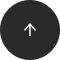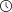- 5 minutes read

# SQL Window Functions Cheat Sheet

Download this 2-page SQL Window Functions Cheat Sheet in PDF or PNG format, print it out, and stick to your desk.

The SQL Window Functions Cheat Sheet provides you with the syntax of window functions, a list of window functions, and examples. You can download this cheat sheet as follows: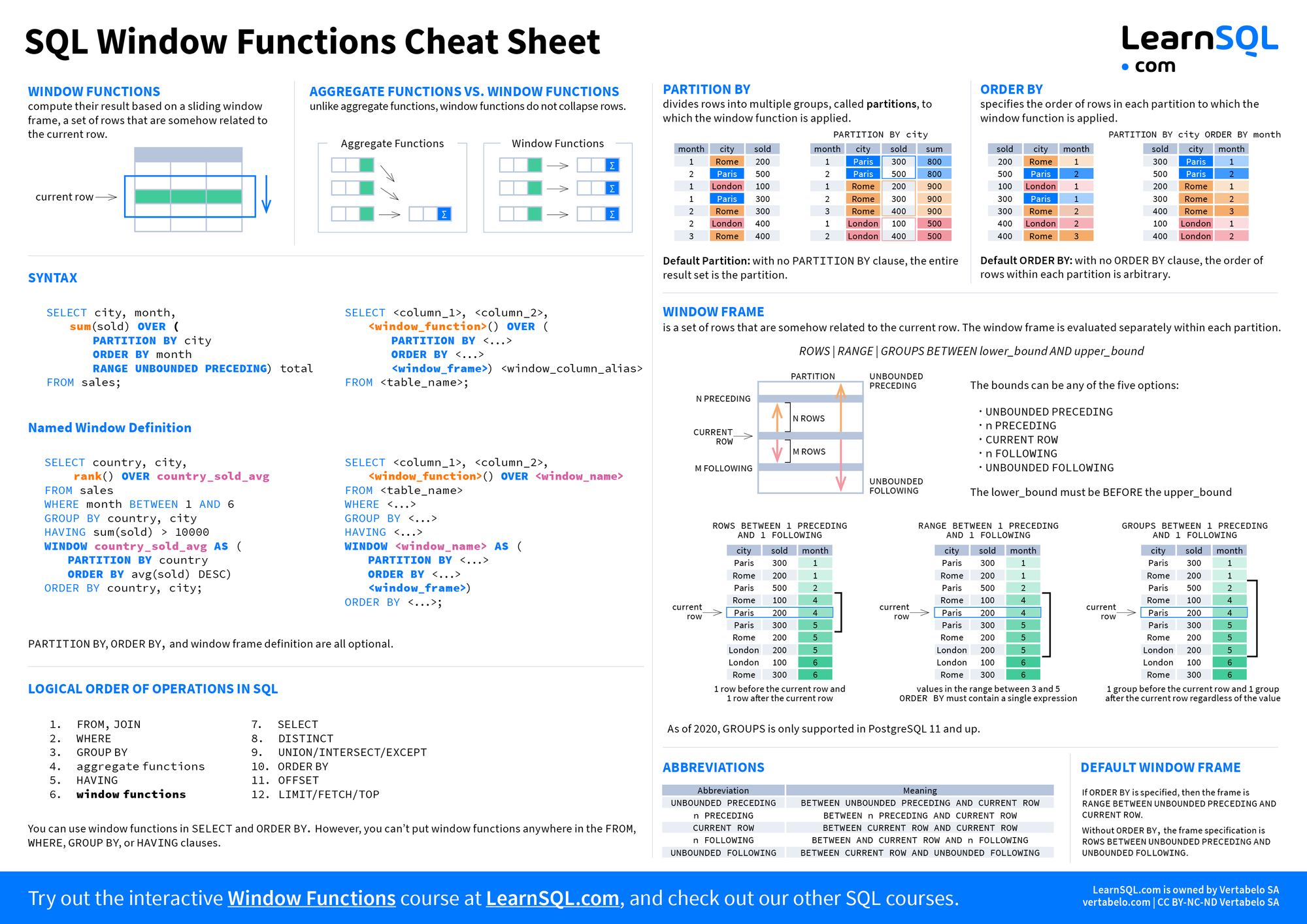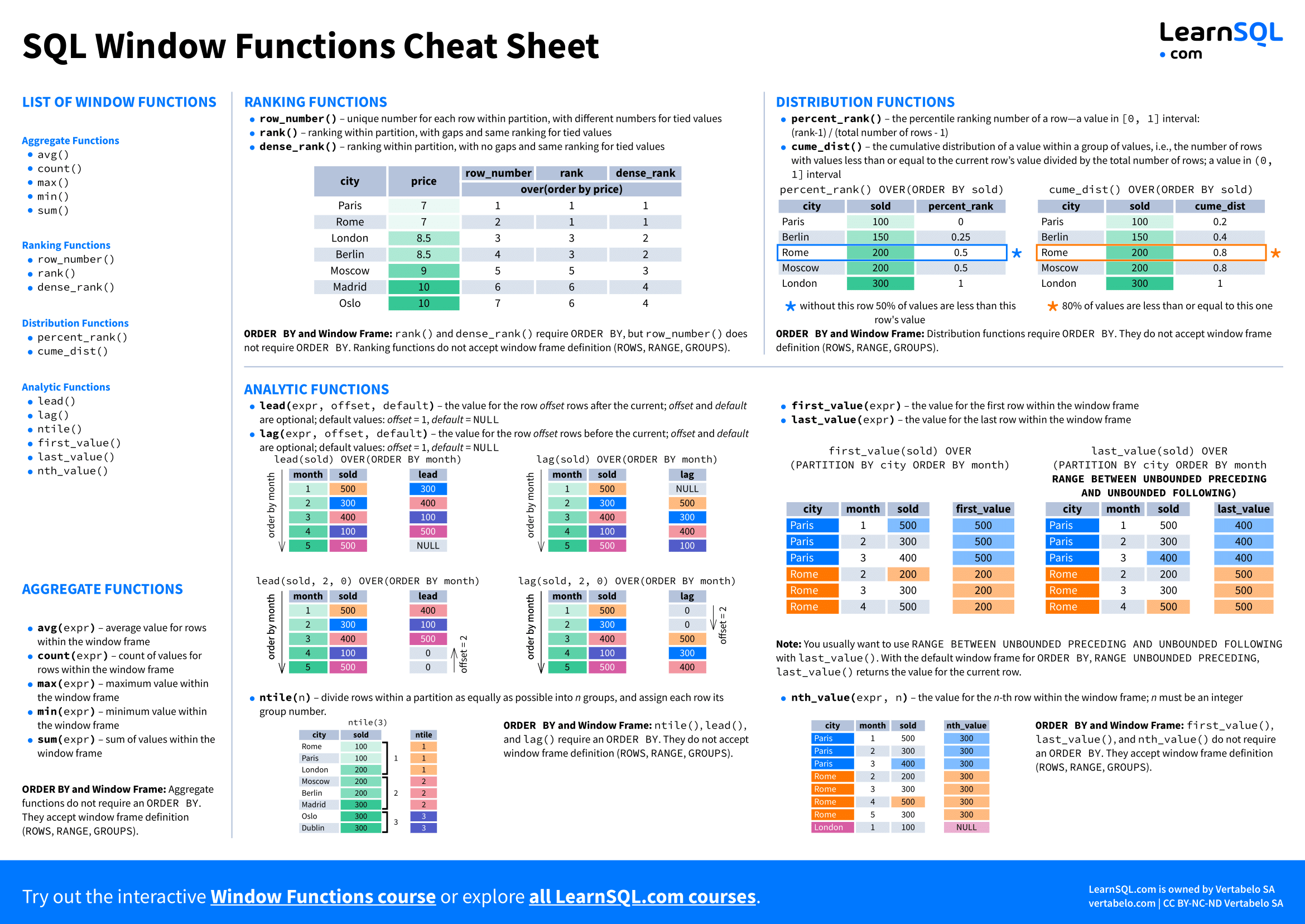## Window Functions

Window functions compute their result based on a sliding window frame, a set of rows that are somehow related to the current row.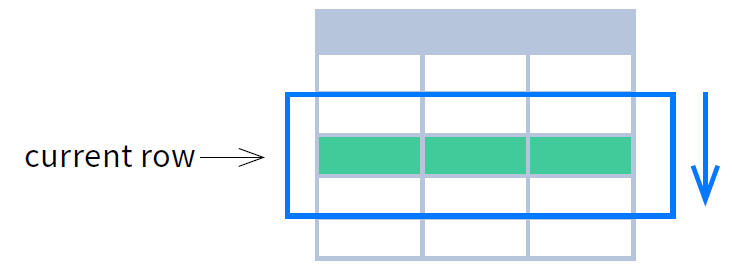## Aggregate Functions vs. Window Functions

Unlike aggregate functions, window functions do not collapse rows.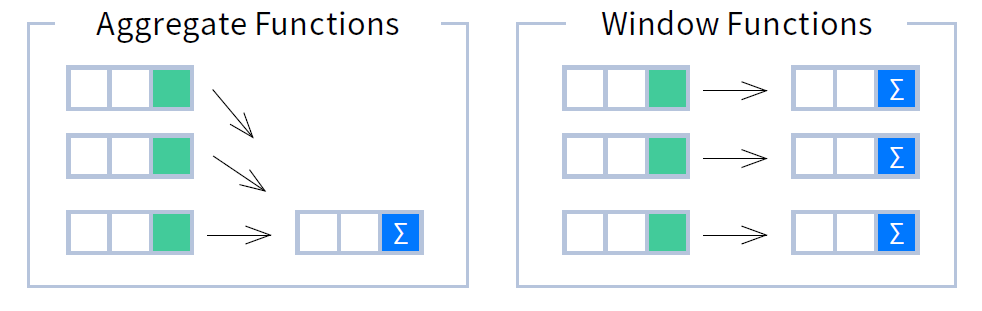## Syntax

```SELECT city, month,
sum(sold) OVER (
PARTITION BY city
ORDER BY month
RANGE UNBOUNDED PRECEDING) total
FROM sales;
```
```SELECT <column_1>, <column_2>,
<window_function> OVER (
PARTITION BY <...>
ORDER BY <...>
<window_frame>) <window_column_alias>
FROM <table_name>;
```

Named Window Definition

```SELECT country, city,
rank() OVER country_sold_avg
FROM sales
WHERE month BETWEEN 1 AND 6
GROUP BY country, city
HAVING sum(sold) > 10000
WINDOW country_sold_avg AS (
PARTITION BY country
ORDER BY avg(sold) DESC)
ORDER BY country, city;
```
```SELECT <column_1>, <column_2>,
<window_function>() OVER <window_name>
FROM <table_name>
WHERE <...>
GROUP BY <...>
HAVING <...>
WINDOW <window_name> AS (
PARTITION BY <...>
ORDER BY <...>
<window_frame>)
ORDER BY <...>;
```

`PARTITION BY`, `ORDER BY`, and window frame definition are all optional.

## PARTITION BY

`PARTITION BY` divides rows into multiple groups, called partitions, to which the window function is applied.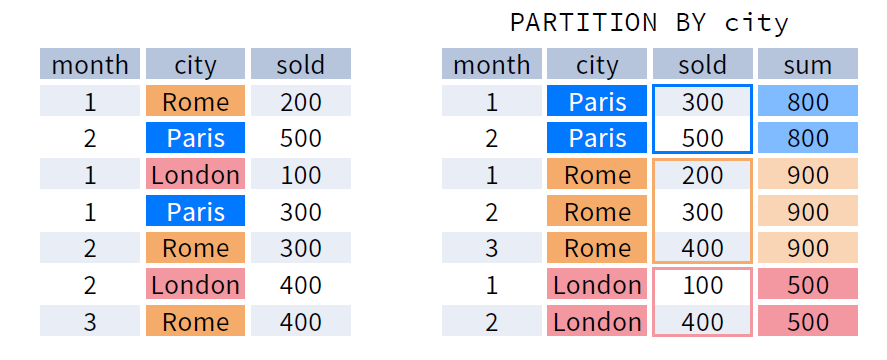Default Partition: With no `PARTITION BY` clause, the entire result set is the partition.

## ORDER BY

ORDER BY specifies the order of rows in each partition to which the window function is applied.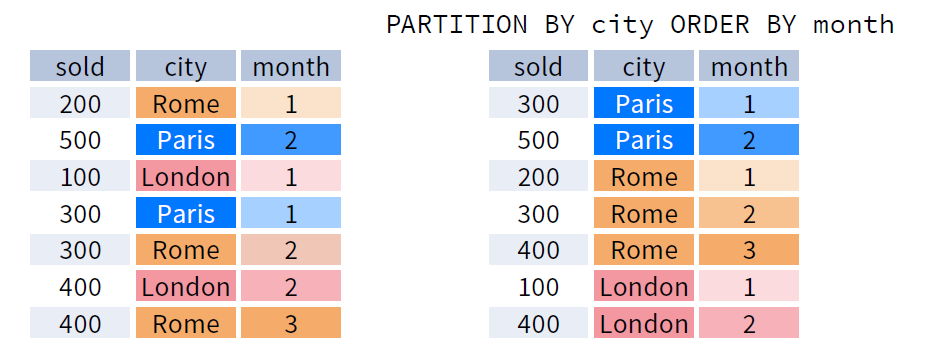Default ORDER BY: With no `ORDER BY` clause, the order of rows within each partition is arbitrary.

## Window Frame

A window frame is a set of rows that are somehow related to the current row. The window frame is evaluated separately within each partition.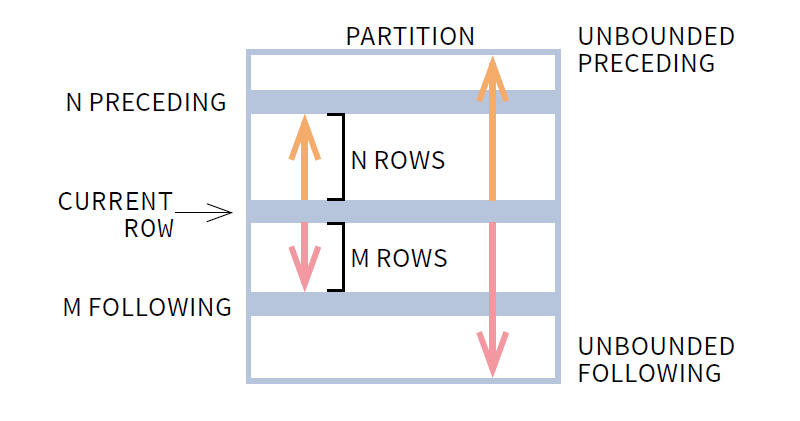```ROWS | RANGE | GROUPS BETWEEN lower_bound AND upper_bound
```

The bounds can be any of the five options:

• `UNBOUNDED PRECEDINGi`
• `n PRECEDING`
• `CURRENT ROW`
• `n FOLLOWING`
• `UNBOUNDED FOLLOWING`

The `lower_bound` must be BEFORE the `upper_bound`.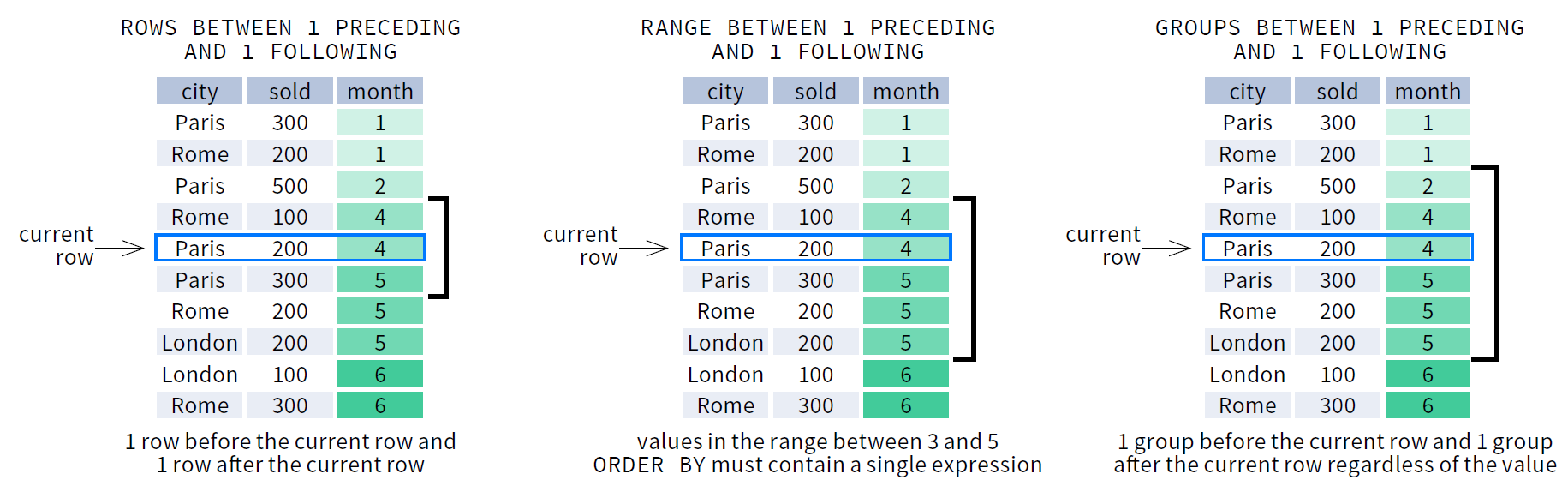As of 2020, `GROUPS` is only supported in PostgreSQL 11 and up.

Abbreviations

AbbreviationMeaning
`UNBOUNDED PRECEDING``BETWEEN UNBOUNDED PRECEDING AND CURRENT ROW`
`n PRECEDING``BETWEEN n PRECEDING AND CURRENT ROW`
`CURRENT ROW``BETWEEN CURRENT ROW AND CURRENT ROW`
`n FOLLOWING``BETWEEN AND CURRENT ROW AND n FOLLOWING`
`UNBOUNDED FOLLOWING``BETWEEN CURRENT ROW AND UNBOUNDED FOLLOWING`

Default Window Frame

• If `ORDER BY` is specified, then the frame is `RANGE BETWEEN UNBOUNDED PRECEDING AND CURRENT ROW`.
• Without `ORDER BY`, the frame specification is `ROWS BETWEEN UNBOUNDED PRECEDING AND UNBOUNDED FOLLOWING`.

## Logical Order of Operations in SQL

1. `FROM`, `JOIN`
2. `WHERE`
3. `GROUP BY`
4. aggregate functions
5. `HAVING`
6. window functions
7. `SELECT`
8. `DISTINCT`
9. `UNION`/`INTERSECT`/`EXCEPT`
10. `ORDER BY`
11. `OFFSET`
12. `LIMIT`/`FETCH`/`TOP`

You can use window functions in `SELECT` and `ORDER BY`. However, you can't put window functions anywhere in the `FROM`, `WHERE`, `GROUP BY`, or `HAVING` clauses.

# List of Window Functions

• Ranking Functions
• `row_number()`
• `rank()`
• `dense_rank()`
• Distribution Functions
• `percent_rank()`
• `cume_dist()`
• Analytic Functions
• `lead()`
• `lag()`
• `ntile()`
• `first_value()`
• `last_value()`
• `nth_value()`
• Aggregate Functions
• `avg()`
• `count()`
• `max()`
• `min()`
• `sum()`

## Ranking Functions

• `row_number()` - unique number for each row within partition, with different numbers for tied values
• `rank()` - ranking within partition, with gaps and same ranking for tied values
• `dense_rank()` - ranking within partition, with no gaps and same ranking for tied values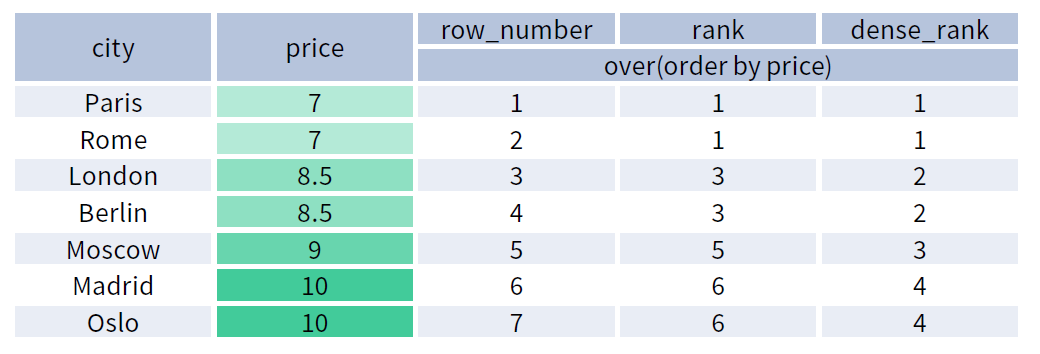ORDER BY and Window Frame: `rank()` and `dense_rank()` require `ORDER BY`, but `row_number()` does not require `ORDER BY`. Ranking functions do not accept window frame definition (`ROWS`, `RANGE`, `GROUPS`).

## Distribution Functions

• `percent_rank()` - the percentile ranking number of a row—a value in [0, 1] interval: (rank-1) / (total number of rows - 1)
• `cume_dist()` - the cumulative distribution of a value within a group of values, i.e., the number of rows with values less than or equal to the current row’s value divided by the total number of rows; a value in (0, 1] interval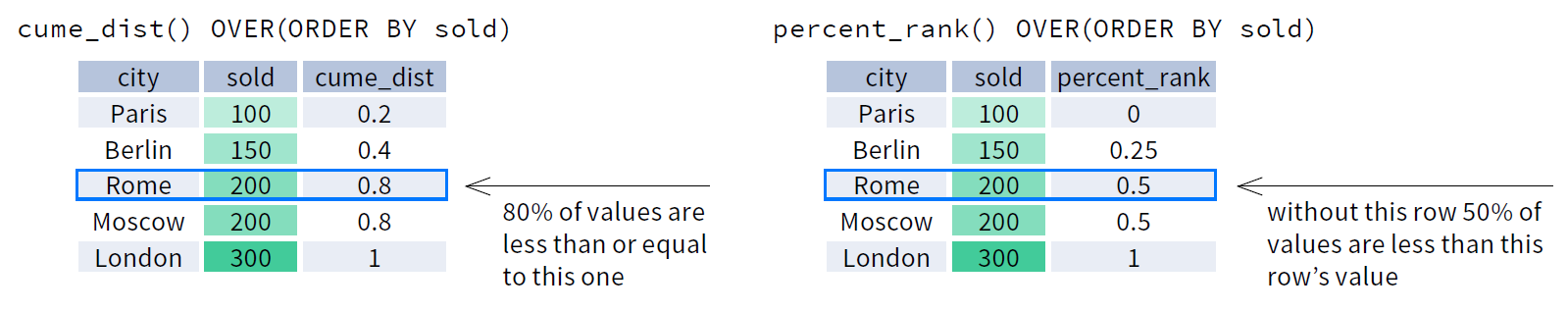ORDER BY and Window Frame: Distribution functions require `ORDER BY`. They do not accept window frame definition (`ROWS`, `RANGE`, `GROUPS`).

## Analytic Functions

• `lead(expr, offset, default)` - the value for the row offset rows after the current; offset and default are optional; default values: offset = 1, default = `NULL`
• `lag(expr, offset, default)` - the value for the row offset rows before the current; offset and default are optional; default values: offset = 1, default = `NULL`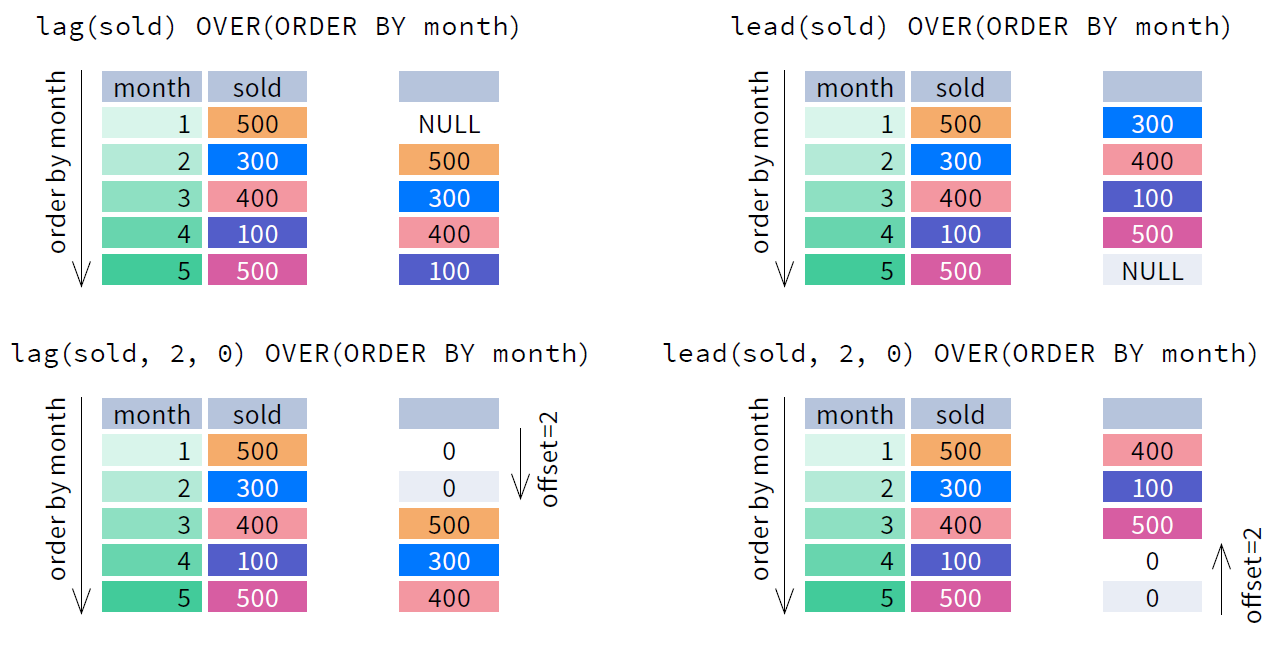• `ntile(n)` - divide rows within a partition as equally as possible into n groups, and assign each row its group number.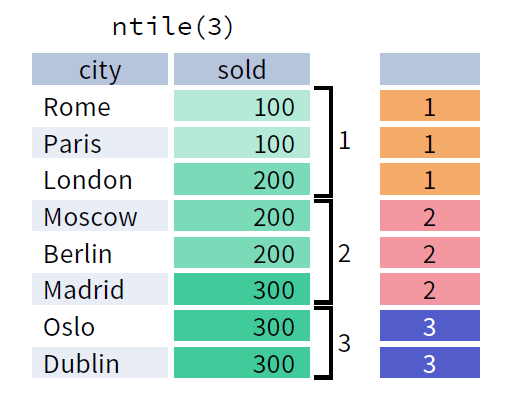ORDER BY and Window Frame: `ntile()`, `lead()`, and `lag()` require an `ORDER BY`. They do not accept window frame definition (`ROWS`, `RANGE`, `GROUPS`).

• `first_value(expr)` - the value for the first row within the window frame
• `last_value(expr)` - the value for the last row within the window frame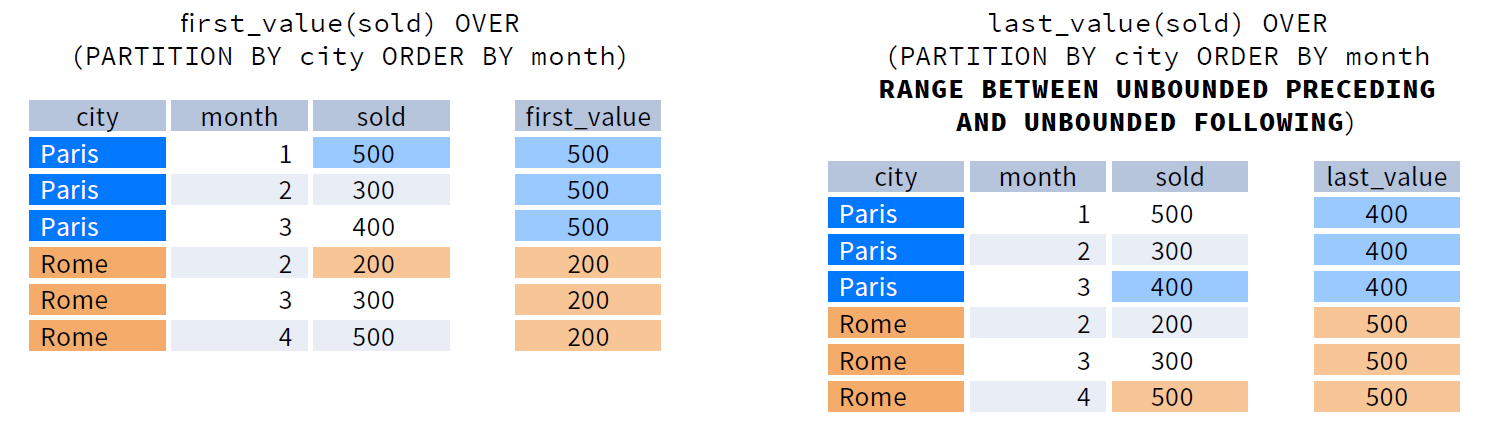Note: You usually want to use `RANGE BETWEEN UNBOUNDED PRECEDING AND UNBOUNDED FOLLOWING` with `last_value()`. With the default window frame for `ORDER BY`, `RANGE UNBOUNDED PRECEDING`, `last_value()` returns the value for the current row.

• `nth_value(expr, n)` - the value for the n-th row within the window frame; n must be an integer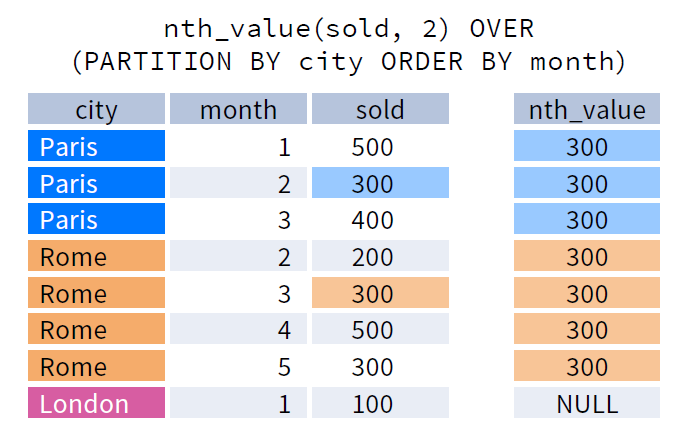ORDER BY and Window Frame: `first_value()`, `last_value()`, and `nth_value()` do not require an `ORDER BY`. They accept window frame definition (`ROWS`, `RANGE`, `GROUPS`).

## Aggregate Functions

• `avg(expr)` - average value for rows within the window frame
• `count(expr)` - count of values for rows within the window frame
• `max(expr)` - maximum value within the window frame
• `min(expr)` - minimum value within the window frame
• `sum(expr)` - sum of values within the window frame

ORDER BY and Window Frame: Aggregate functions do not require an `ORDER BY`. They accept window frame definition (`ROWS`, `RANGE`, `GROUPS`).